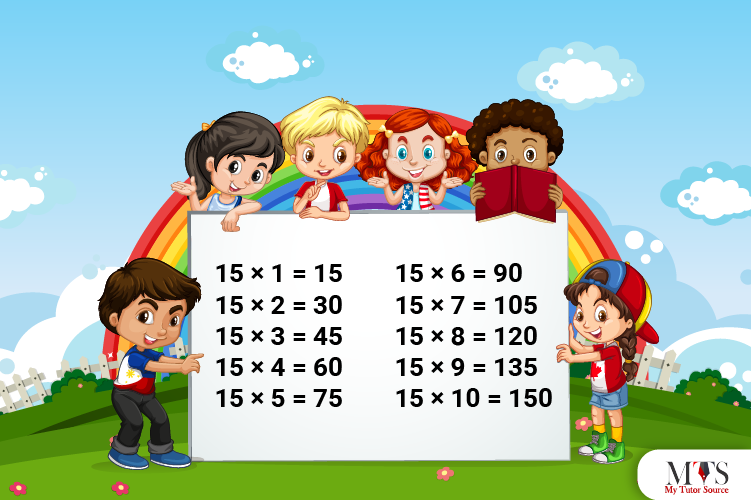# Multiplication Table of 15 – Tips to Memorize 15 Times Table & Example QuestionsTo improve problem-solving skills and establish a core understanding of the concept of numbers and their complexity, all professional teachers and math tutors prefer teaching multiplication tables. For better grades, greater confidence, and enhancement of knowledge, let us learn the multiplication table of 15. Maths comes with tips, tricks, and patterns, so the multiplication table of 15 is. Go through this article and discover tips for quick learning and how to perform calculations using the table of 15.

## Table of 15

Below we have provided the table of 15 up to its 20 multipliers. Memorizing it by heart will enable you to perform calculations within seconds and confidently.

15 x 1 = 15
15 x 2 = 30
15 x 3 = 45
15 x 4 = 60
15 x 5 = 75
15 x 6 = 90
15 x 7 = 105
15 x 8 = 120
15 x 9 = 135
15 x 10 = 150
15 x 11 = 165
15 x 12 = 180
15 x 13 = 195
15 x 14 = 210
15 x 15 = 225
15 x 16 = 240
15 x 17 = 255
15 x 18 = 270
15 x 19 = 285
15 x 20 = 300

## Method to Read Table of 15

This is how we read the table of 15:

Fifteen ones are fifteen (15)
Fifteen twos are thirty (30)
Fifteen threes are forty-five (45)
Fifteen fours are sixty (60)
Fifteen fives are seventy-five (75)
Fifteen sixes are ninety (90)
Fifteen sevenths are one hundred and five (105)
Fifteen eighths are one hundred and twenty (120)
Fifteen nines are one hundred and thirty-five (135)
Fifteen tens are one hundred and fifty (150)
Fifteen elevens are one hundred and sixty-five (165)
Fifteen twelves are one hundred and eighty (180)
Fifteen thirteens are one hundred and ninety-five (195)
Fifteen fourteens are two hundred and ten (210)
Fifteen fifteenths are two hundred and twenty-five (225)
Fifteen sixteenths are two hundred and forty (240)
Fifteen seventeens are two hundred and fifty-five (255)
Fifteen eighteenths are two hundred and seventy (270)
Fifteen nineteens are two hundred and eighty-five (285)
Fifteen twenties are three hundred (300)

## Tips for Memorizing Multiplication Table of 15

To make a learning multiplication table of 15 less daunting, we have covered a few tips to learn it quickly. Go through the points given below and pick a tip that suits your learning style better:

### Tip # 1: Ten Place Digit Pattern

The pattern of the results of the 15 times table is unique yet interesting compared to other multiplication tables of mathematics. The tens place of the product of the 15 times table is two consecutive odd natural numbers and two consecutive even natural numbers.

For example:

15 × 1 = 15 (digit at ten’s place is 1, odd number)
15 × 2 = 30 (digit at ten’s place is 3, odd number)
15 × 3 = 45 (digit at ten’s place is 4, even number)
15 × 4 = 60 (digit at ten’s place is 6, even number)
15 x 5 = 75 (digit at ten’s place is 7, odd number)
15 x 6 = 90 (digit at ten’s place is 9, odd number)

and so on…

### Tip # 2: Unit Place Digit Pattern

One of the easiest and quick ways to memorize and figure out if a certain number is divisible by 15 times table or not is to check its unit place digits. 15 times tables always have either 5 or 0 at their unit place.

### Tip # 3: Repeated Addition

Students must know the repeated addition method as plenty of questions in exams demand solutions in this method. To find the multiplier results of the 15 times table, skip the count for 15 or add the previous result in 15.

### Example Question of Table of 15

Question: If 11 pots have 15 flowers each, then how many flowers are there in total? Use the repeated addition method to find the answer.

Solution:

Given that,
Total Pots = 11
Flowers in each pot = 15
15 + 15 + 15 + 15 + 15 + 15 + 15 + 15 + 15 + 15 + 15 = 165
Also,
11 times 15 = 165
Hence, there are a total of 165 flowers.

Question: Find the 9th multiple of 15?

Solution:

Given that,
9 multiple of 15
Mathematically it is written as,
9 x 15 = 285
Thus, the 9th multiple of 15 is 285.

Question: Using table of 14, find 15 times 4 plus 15 times 7?

Solution:

Given that,
15 times 4 plus 15 times 7
In mathematical form,
= 15 x 4 + 15 x 7
= 75 + 105
= 180
Hence, 15 times 4 plus 15 times 7 is 180.

Question: Mack wants to buy 2 cupcakes for each of his 15 friends. Using the table of 15, how many cupcakes should Mack buy, if each friend will get 2 cupcakes.

Solution:

Given that,
Total friends = 15
Cupcakes for each friend = 2
Using 15 times table,
= 15 x 2 = 30
Thus, Mack has to buy 30 cupcakes.

Question: What is 15 times 5 minus 5?

Solution:

Given that,
15 times 5 minus 5
In mathematical form,
= 15 x 5 – 5
= 75 – 5
= 70
Hence, 15 times 5 minus 5 is 70.

### Find Top Tutors in Your AreaWith over 3 years of experience in teaching, Chloe is very deeply connected with the topics that talk about the educational and general aspects of a student's life. Her writing has been very helpful for students to gain a better understanding of their academics and personal well-being. I’m also open to any suggestions that you might have! Please reach out to me at chloedaniel402 [at] gmail.com